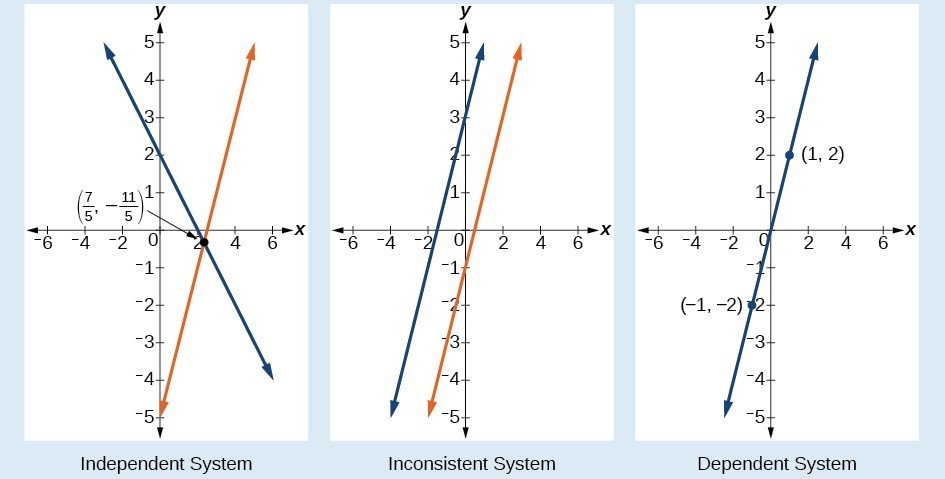## Solutions of Systems Overview

### Learning Outcomes

• Identify the three types of solutions possible from a system of two linear equations.
• Use a graph to find solution(s) to a system of two linear equations.

In order to investigate situations such as that of the skateboard manufacturer, we need to recognize that we are dealing with more than one variable and likely more than one equation. A system of linear equations consists of two or more linear equations made up of two or more variables such that all equations in the system are considered simultaneously. To find the unique solution to a system of linear equations, we must find a numerical value for each variable in the system that will satisfy all equations in the system at the same time. Some linear systems may not have a solution and others may have an infinite number of solutions. In order for a linear system to have a unique solution, there must be at least as many equations as there are variables. Even so, this does not guarantee a unique solution.

In this section, we will look at systems of linear equations in two variables, which consist of two equations that contain two different variables. For example, consider the following system of linear equations in two variables.

\begin{align}2x+y&=15\\[1mm] 3x-y&=5\end{align}

The solution to a system of linear equations in two variables is any ordered pair that satisfies each equation independently. In this example, the ordered pair $(4,7)$ is the solution to the system of linear equations. We can verify the solution by substituting the values into each equation to see if the ordered pair satisfies both equations. Shortly we will investigate methods of finding such a solution if it exists.

\begin{align}2\left(4\right)+\left(7\right)&=15 &&\text{True} \\[1mm] 3\left(4\right)-\left(7\right)&=5 &&\text{True} \end{align}

In addition to considering the number of equations and variables, we can categorize systems of linear equations by the number of solutions. A consistent system of equations has at least one solution. A consistent system is considered to be an independent system if it has a single solution, such as the example we just explored. The two lines have different slopes and intersect at one point in the plane. A consistent system is considered to be a dependent system if the equations have the same slope and the same y-intercepts. In other words, the lines coincide so the equations represent the same line. Every point on the line represents a coordinate pair that satisfies the system. Thus, there are an infinite number of solutions.

Another type of system of linear equations is an inconsistent system, which is one in which the equations represent two parallel lines. The lines have the same slope and different y-intercepts. There are no points common to both lines; hence, there is no solution to the system.

### A General Note: Types of Linear Systems

There are three types of systems of linear equations in two variables, and three types of solutions.

• An independent system has exactly one solution pair $\left(x,y\right)$. The point where the two lines intersect is the only solution.
• An inconsistent system has no solution. Notice that the two lines are parallel and will never intersect.
• A dependent system has infinitely many solutions. The lines are coincident. They are the same line, so every coordinate pair on the line is a solution to both equations.

Below is a comparison of graphical representations of each type of system.### How To: Given a system of linear equations and an ordered pair, determine whether the ordered pair is a solution.

1. Substitute the ordered pair into each equation in the system.
2. Determine whether true statements result from the substitution in both equations; if so, the ordered pair is a solution.

### Example: Determining Whether an Ordered Pair Is a Solution to a System of Equations

Determine whether the ordered pair $\left(5,1\right)$ is a solution to the given system of equations.

\begin{align}x+3y&=8\\ 2x-9&=y \end{align}

### Try It

Determine whether the ordered pair $\left(8,5\right)$ is a solution to the following system.

$\begin{gathered}5x - 4y=20\\ 2x+1=3y\end{gathered}$

## Solving Systems of Equations by Graphing

There are multiple methods of solving systems of linear equations. For a system of linear equations in two variables, we can determine both the type of system and the solution by graphing the system of equations on the same set of axes.

### Example: Solving a System of Equations in Two Variables by Graphing

Solve the following system of equations by graphing. Identify the type of system.

\begin{align}2x+y&=-8\\ x-y&=-1\end{align}

### Try It

Solve the following system of equations by graphing.

$\begin{gathered}2x - 5y=-25 \\ -4x+5y=35 \end{gathered}$

### Q&A

#### Can graphing be used if the system is inconsistent or dependent?

Yes, in both cases we can still graph the system to determine the type of system and solution. If the two lines are parallel, the system has no solution and is inconsistent. If the two lines are identical, the system has infinite solutions and is a dependent system.

### Try IT

Plot the three different systems with an online graphing tool. Categorize each solution as either consistent or inconsistent. If the system is consistent determine whether it is dependent or independent. You may find it easier to plot each system individually, then clear out your entries before you plot the next.
1)
$5x-3y = -19$
$x=2y-1$

2)
$4x+y=11$
$-2y=-25+8x$

3)
$y = -3x+6$
$-\frac{1}{3}y+2=x$

## Contribute!

Did you have an idea for improving this content? We’d love your input.